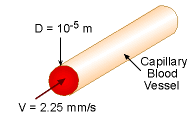Ch 6. Modeling and Similitude Multimedia Engineering Fluids DimensionalAnalysis Similitude DimensionlessGroups
 Chapter 1. Basics 2. Fluid Statics 3. Kinematics 4. Laws (Integral) 5. Laws (Diff.) 6. Modeling/Similitude 7. Inviscid 8. Viscous 9. External Flow 10. Open-Channel Appendix Basic Math Units Basic Equations Water/Air Tables Sections Search eBooks Dynamics Fluids Math Mechanics Statics Thermodynamics Author(s): Chean Chin Ngo Kurt Gramoll ©Kurt GramollFLUID MECHANICS - CASE STUDY SOLUTIONBlood Flow in Vessel The Reynolds number is given by      Re = ρVL/μ where L is the characteristic length. It is assumed that the blood vessel is not collapsible, which means the vessel wall is not deformable, and the stenosis scenario (i.e., constriction or narrowing of coronary vessels due to the buildup of fat and cholesterol) is not considered. Since the blood vessel maintains the circular cross section, the diameter (D) is chosen as the characteristic length, which is 10-5 m. At body temperature, the density (ρ) and viscosity (μ) of the plasma is given as 980 kg/m3 and 0.0014 N-s/m2, respectively. Since the plasma consists 90% of water, the density and viscosity of plasma are close to thase of water (ρ = 999 kg/m3 and μ = 0.0011 N-s/m2). The Reynolds number is calculated to be      Re = (980)(2.25)(10-3)(10-5)/(0.0014)           = 0.0158 Based on the pipe flow model, the calculated Reynolds number is less than 2,100, hence the flow is laminar.

Practice Homework and Test problems now available in the 'Eng Fluids' mobile app
Includes over 250 problems with complete detailed solutions.
Available now at the Google Play Store and Apple App Store.